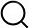# Also in the Article

Mechanism of large-scale flow reversals in turbulent thermal convection

Procedure

Figure S3 shows the experimental setup of the shadowgraph measurement. A high-quality white light-emitting diode (LED) (LUXEON S1000, Philips Lightning) equipped with a cooling fan was used as a stable white light source. The LED was powered by a DC power supply (PL303, TTi) with a long-term stability of ±1 mA. The working voltage and current of the LED are 25.50 V and 350 mA, respectively, which correspond to a working power of ~9 W. The while light was guided by an optical fiber to a 1-mm-diameter pinhole, which was positioned at the back focal point of a 250-mm-diameter convex lens so that a wide collimating beam of white light is incident normally to the entire circular section of the convection cell. The shadowgraph image was projected onto a white paper screen at a distance z1 = 800 mm away from the back window of the convection cell. A charge-coupled device (CCD) camera with a spatial resolution of 960 × 960 pixels was used to record movies of the shadowgraph images at a sampling rate of 10 frames per second.

Figure S4A shows the measured background image I0(x, y) of a static (nonconvecting) fluid (water) when there is no temperature difference applied across the top and bottom conducting plates. While the two flat circular windows of the cell are made of polished Plexiglas plates and no visible scratch is found by the naked eye, one can observe residual circular marks in the shadowgraph resulting from the machine work on the Plexiglas surface. Figure S4B shows a typical shadowgraph image I(x, y, t) of a convecting fluid (water) when a temperature difference ΔT = 25.2°C is applied across the convection cell. The convecting fluid contains many cold and warm thermal plumes, which bend the parallel light rays to regions with higher values of the refractive index n per Huygens’s principle and form a pattern with bright and dark strips on the observing screen.

Assuming that each image has N pixels, the 2D average of the normalized intensity ζ(x, y, t) in Eq. 1 over the cross-sectional area A can be carried out by(7)where i is the pixel label within the bright field. Because the convection cell is thin and thus the thermal plumes do not overlap very much along the optical path W, the 2D average in Eq. 7 is equivalent to a 3D volume average over the entire convecting fluid. In this case, we have (28, 29)(8)where γ = dn/dT. In the experiment, we recorded movies of the shadowgraph images both with and without a temperature difference ΔT across the cell and used Eq. 7 to obtain the time series data ζ(t) for three convecting fluids at different Rayleigh numbers. At a fixed value of Ra, each movie lasts for 16 hours and thus contains 5.76 × 105 shadowgraph images in total.

With the special design of the thin disc cell, the volume average 〈…〉V in Eq. 8 can be rewritten as(9)where J = −k||T is the local conductive heat flux across the circular sidewall S0 of the convection cell. Here, the cell volume is V = π(D/2)2W and the surface area of each conducting plate is S1 = (πD/3)W. With Eqs. 8 and 9, we have(10)where(11)is the net accumulation or loss of heat flux across the entire convecting fluid. Above, Jb is the heat flux flowing into the system from the bottom conducting plate, and Jt is the heat flux going out of the system from the top conducting plate. All other parts of the cell are assumed to be adiabatic, and thus, they do not contribute to δJ(t). Because γ is a negative number, ζ(t) and δJ(t) have opposite signs.

With the measured heat flux J0, Eq. 10 can be rewritten in a dimensionless form(12)Equation 12 thus provides a sensitive null test on whether the net heat flux passing through the convection cell is balanced or not.

Q&A